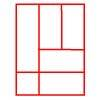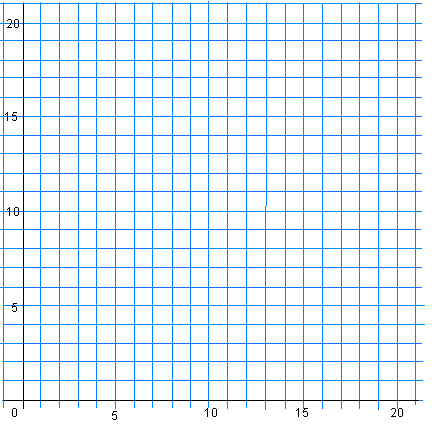#### You may also like### Bracelets

Investigate the different shaped bracelets you could make from 18 different spherical beads. How do they compare if you use 24 beads?### Cut and Make

Cut a square of paper into three pieces as shown. Now,can you use the 3 pieces to make a large triangle, a parallelogram and the square again?### Is a Square a Rectangle?

How many rectangles can you find in this shape? Which ones are differently sized and which are 'similar'?

# A Cartesian Puzzle

## A Cartesian Puzzle

Here are the coordinates of some quadrilaterals, but in each case one coordinate is missing!  The coordinates are given going round each quadrilateral in an anti-clockwise direction.

1. $(2,11), \; (0,9),\; (2,7),\; (?,?)$
2. $(3,7),\; (3,4),\; (8,4),\; (?,?)$
3. $(18,3),\; (16,5), \;(12,5),\; (?,?)$
4. $(13,12),\; (15,14),\; (12,17),\; (?,?)$
5. $(7,14),\; (6,11),\; (7,8),\; (?,?)$
6. $(15,9),\; (19,9),\; (16,11),\; (?,?)$
7. $(11,3),\; (15,2),\; (16,6),\; (?,?)$
8. $(9,16),\; (2,9),\; (9,2),\; (?,?)$

The quadrilaterals are all symmetrical. They may have rotational symmetry, or line symmetry, or both.

Can you work out what the missing coordinates are if you know they are all positive? Is there more than one way to find out?

Now plot those eight missing coordinates on a graph like the one below.
What shape do they make and what sort of symmetry does it have?### Why do this problem?

This problem is one that requires some familiarity with coordinates in the first quadrant. It will also call on knowledge of both rotational and line symmetry, and properties of various quadrilaterals.

### Possible approach

You could play a game of 'twenty questions' to begin with so that pupils get a chance to familiarise themselves with properties of shapes. Choose a quadrilateral and write the name of it on a piece of paper. Invite the class to ask questions to guess what your quadrilateral is, but you can only answer yes or no. Keep a tally of the number of questions asked - if they get it in fewer than twenty, they win, otherwise you win. You could repeat this a few times with pupils choosing shapes.

You could start on the problem itself by showing it to the group on an interactive whiteboard. Alternatively, if learners are already very familiar with coordinates in the first quadrant, you could invite them to work in pairs from a printed sheet of the problem from the beginning. It is important that they are able to talk through their ideas with a partner while doing the problem. (This sheet of the first quadrant could be used for both rough working and the final results.) Otherwise supply plenty of squared paper!

One of the nice things about this problem is that learners will know that they have solved it correctly. In the plenary, therefore, you can concentrate on asking some pairs to explain the way they tackled the problem, rather than focusing on the answer. Were some ways more efficient than others?

### Key questions

What kind of quadrilateral do you think this one is?
Where is its fourth vertex?
What kind of symmetry do you think this quadrilateral has? How do you know?

### Possible support

You might want to tell some children that the shapes include one parallelogram, one trapezium and one rhombus, and are otherwise squares and rectangles.

### Possible extension

Learners could plot their own quadrilaterals with one vertex of each forming a hexagon and so make a similar problem for a friend to try.